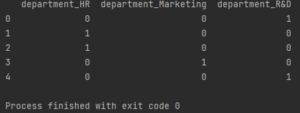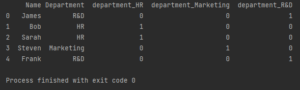Pandas How To Uncategorized How to one hot encode a column in Pandas

# How to one hot encode a column in Pandas

Call me a geek, but I like my data much better in the form of zeros and ones. In this post you will learn how to one hot encode a column in Pandas.

## How to one hot encode a column in Pandas

To encode a column of data in Pandas, simply use the built-in get_dummies function.

Here is my sample dataframe.

```import pandas as pd

my_df = pd.DataFrame({'Name': ['James','Bob','Sarah','Steven','Frank'],
'Department': ['R&D','HR','HR','Marketing','R&D']})

print(my_df)
```

As you can see the departments repeat themselves. So it will encode the department column.

```import pandas as pd

my_df = pd.DataFrame({'Name': ['James','Bob','Sarah','Steven','Frank'],
'Department': ['R&D','HR','HR','Marketing','R&D']})

my_df = pd.get_dummies(my_df['Department'], prefix='department')

print(my_df)

```

I used the get_dummies function and just entered the column name as a parameter. As an additional parameter, I indicated how the name of the columns that will be created in the new dataframe should begin.

This name is important because in this way you can easily spoil the readability of the data. The column name must make it clear what the zeros and ones in that column mean.Three new columns were created to replace one departmental column. Now instead of department names my dataframe consists of zeros and ones. A one in the department_R&D column means that there is an employee working in R&D under the given index.

## How to add one hot encode a column to dataframe

Alternatively, if you need encoding but don’t want to get rid of the existing name column, you can combine both approaches. To add encoding to the dataframe, use the concat function.

```import pandas as pd

my_df = pd.DataFrame({'Name': ['James','Bob','Sarah','Steven','Frank'],
'Department': ['R&D','HR','HR','Marketing','R&D']})

my_df = pd.concat([my_df,pd.get_dummies(my_df['Department'], prefix='department')], axis=1)

print(my_df)

```As you can see, thanks to the concat function, I combined the existing dataframe with new columns containing encodings.

Get_dummies documentation
Concat documentation
How to replace values in a column
How to Sort Data Frame
How to get column names

## How to drop all columns except one in PandasHow to drop all columns except one in Pandas

Here is a tutorial on how to drop all columns except one in Pandas.

## How to filter by column valueHow to filter by column value

Here’s the tutorial on how to filter by column value. I show several ways to filter columns in Pandas.

## How to join two dataframes on keyHow to join two dataframes on key

You can join two pandas DataFrames on a common key column by specifying the key column in the on parameter of the merge method. Here’s an example of how to